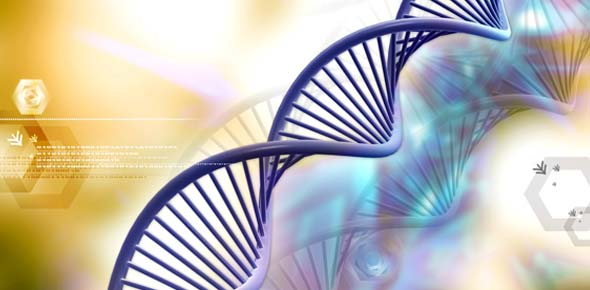Molecular Basis Of Inheritance

15 Questions | Total Attempts: 1488SettingsTime: 30 Minute

• 1.
Select the incorrect option for genetic code?
• A.

It is nearly universal

• B.

It is degenerate

• C.

It is unambiguous

• D.

A codon in mRNA is read in a non contiguous fashion

• 2.
Removal of introns and joining the exons in a defined order in a transcription unit is called
• A.

Tailing

• B.

Transformation

• C.

Capping

• D.

Splicing

• 3.
Discontinuous synthesis of DNA occurs in one strand, because:
• A.

DNA molecule being synthesized is very long

• B.

DNA dependent DNA polymerase catalyses polymerization only in one direction (5' - 3')

• C.

It is a more efficient process

• D.

DNA ligase has to have a role

• 4.
Who amongst the following scientists had no contribution in the development of the double helix model for the structure of DNA?
• A.

Rosalind Francklin

• B.

Maurice Wilkins

• C.

Erwin Chargaff

• D.

Meselson and Stahl

• 5.
Which of the following steps in transcription is catalysed by RNA polymerase?
• A.

Initiation

• B.

Elongation

• C.

Termination

• D.

All of the above

• 6.
Which of the following are the functions of RNA?
• A.

It is a carrier of genetic information from DNA to ribosomes synthesizing polypetides

• B.

It carries amino acids to ribosomes

• C.

It is a constituent component of ribosomes

• D.

All of the above

• 7.
In some viruses, DNA is synthesized by using RNA as template. Such a DNA is called:
• A.

A-DNA

• B.

B-DNA

• C.

CDNA

• D.

RDNA

• 8.
If Meselson and Stahl's experiment is continued for four generations is bacteria, the ratio of 15N/ 15N: 15N/14N: 14 N/14N containing DNA in the fourth generation would be:
• A.

1:1:0

• B.

1:4:0

• C.

0:1:3

• D.

0:1:7

• 9.
If the base sequence of a codon in mRNA is 5' AUG-3', the sequence of tRNA pairing with it must be:
• A.

5' UAC – 3'

• B.

5' - CAU - 3'

• C.

5' -AUG - 3'

• D.

5' - GUA - 3'

• 10.
If the sequence of nitrogen base of the coding strand of DNA in a transcription unit is:
• A.

5' – A U G AA U G - 3'

• B.

5' – U A C U U A C - 3'

• C.

5' – C AU U C A U - 3'

• D.

5' – G U A A G U A - 3'

• 11.
The initiate translation, the mRNA first blinds to:
• A.

The smaller ribosomal sub-unit

• B.

The larger ribosomal sub-unit

• C.

The whole ribosome

• D.

No such specificity exists

• 12.
In E.Coil, the lac Operon gets switched on when:
• A.

Lactose is present and it binds to the repressor

• B.

Repressor binds to operator

• C.

RNA polymerase binds to the operator

• D.

Lactose is present and it binds to RNA polymerase

• 13.
Which of the following sequence will be produced as a result of transcription of the DNA sequence –CGATTACAG
• A.

GCUAAUGUC

• B.

CGUAAUCUG

• C.

GCTAATGTC

• D.

GCUAATCTG

• 14.
Sickle-cell haemoglobin has valine in place of glutamic acid at position 6 in the f3 polypeptide chain. Reason: Sickle-cell anaemia is expressed only in homozygous recessive state.
• A.

If both A and R are true R is the correct explanation of A

• B.

If both A and R are true but R is not the correct explanation of A

• C.

If A is true but R is false

• D.

If both A and R are false

• 15.
DNA fingerprinting is very well known for its application in paternity testing is case of disputes. Reason: It employs the principle of polymorphism in DNA sequences as the polymorphisms are inheritable from parents to children
• A.

If both A and R are true R is the correct explanation of A

• B.

If both A and R are true but R is not the correct explanation of A

• C.

If A is true but R is false

• D.

If both A and R are false

Related TopicsBack to top